# Indeterminate Form

(redirected from Zero raised by zero)

## Indeterminate Form

in mathematics, an expression whose limit cannot be found by direct application of the usual limit theorems. Indeterminate forms may be of the following types: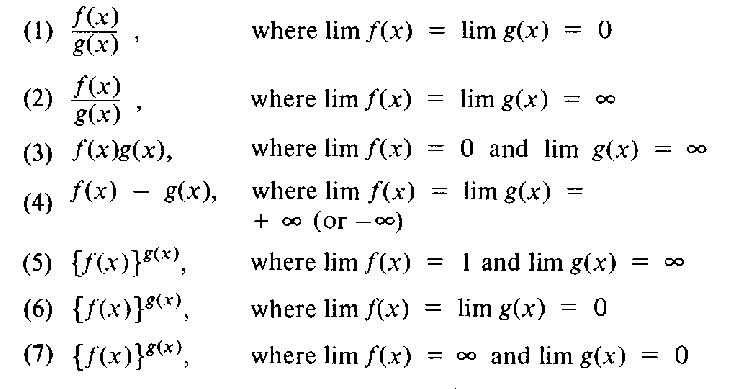Other indeterminate forms are (sin x)/x as x ←, 0, where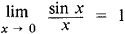and [1 + (1/x)] x as x → ∞, where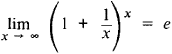(e = 2.71828 ... is the base of the natural logarithms). The seven types of indeterminate forms listed above are denoted as (1) 0/0, (2) ∞/∞, (3) 0 • ∞ ,(4) ∞ -∞ ,(5) 1∞, (6) 0°, (7) ∞ °. We observe that a given function can be an indeterminate form for some values of the independent variable and not for others [for example, the expression (sin x)/x as x x → π is not an indeterminate form]. Not every indeterminate form has a limit. The expression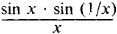as x → 0 does not tend to a limit: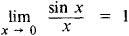but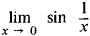does not exist.

Finding the limit of an indeterminate form (if it exists) is often based on replacing a given function by a different function that has the same limit but is not an indeterminate form. This replacement is sometimes achieved by means of algebraic transformations. For example, if we divide the numerator and denominator in the expression (1 − x)/(1 − x2) by 1 − x, then we obtain 1/(1 + x).Therefore,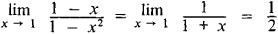Indeterminate forms of types (1) and (2) can often be evaluated by means of l’Hôpital’s rule. L’Hópital’s rule asserts that for indeterminate forms of types (1) and (2) the equation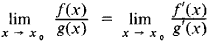holds provided that f(x) and g(x) are differentiable in a neighborhood of x0 (x0 may be a finite point or a point at infinity) with the possible exception of x0, and provided that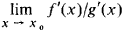exists. Using this rule, we find, for example, that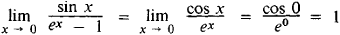It may be that [/”’(*)]/[ g’(x)] is again an indeterminate form of type (1) or (2). L’Hópital’s rule can then be applied once more (if the relevant conditions hold) and so on. This approach, however, is not always successful. For example, application of L’Hópital’s rule to the indeterminate form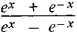[f(x) = ex + e-x and g(x) = ex - e-x] for x →0 pointless. It may also happen that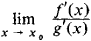does not exist, whereasof type (1) or (2) does. Thus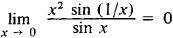whiledoes not exist.

The expansion of functions in series is a powerful method for finding the limits of indeterminate forms. For example, since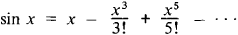we have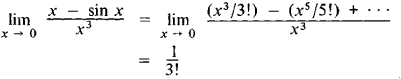Indeterminate forms of types (3)-(7) can be reduced to either type (1) or (2). For example, as x ← π/2,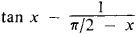is an indeterminate form of type (4). Now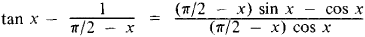and as x − π/2 the latter indeterminate form is of type (1) and has the limit 0. An indeterminate form of type (3) is reduced to an indeterminate form of type (1) or (2) by the transformation f(x) g(x) = f(x)/h(x) or g(x)/k(x), where h (x) = g(x) and k(x) = f(x). Finally, if we denote by u(x) the logarithm of indeterminate forms of types (5), (6), and (7), that is, if we set u(x) = g(x) In f(x), then u(x) is an indeterminate form of type (3), which, as was indicated, can be reduced to an indeterminate form of type (1) or (2). Finally, consider {f (x)}g(x) = eu(x). If we can find the (presumably existing) limit of u(x) then we can also find the limit of the given indeterminate form. For example, for xx as x →0 we have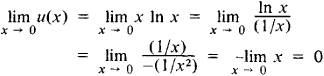and, consequently,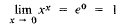### REFERENCES

II’in, V. A. , and E. G. Pozniak. Osnovy matematicheskogo analiza, 3rd ed., part 1. Moscow, 1971.
Kudriavtsev, L. D. Matematicheskii analiz, 2nd ed., vol. 1. Moscow, 1973.
Site: Follow: Share:
Open / Close